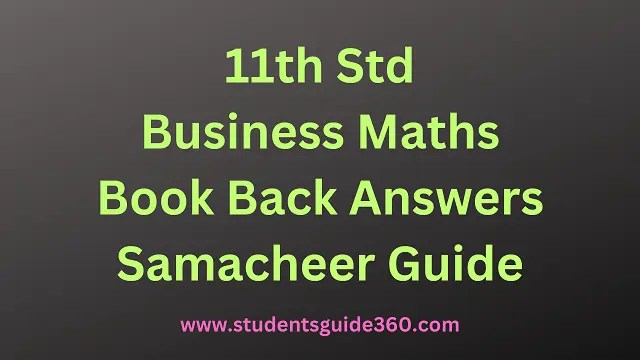### 11th Business Maths Volume 1 – Solutions

#### 11th Business Maths Guide Chapter 1 Matrices and Determinants

• Chapter 1 Matrices and Determinants Exercise 1.1
• Chapter 1 Matrices and Determinants Exercise 1.2
• Chapter 1 Matrices and Determinants Exercise 1.3
• Chapter 1 Matrices and Determinants Exercise 1.4
• Chapter 1 Matrices and Determinants Exercise 1.5

• Chapter 2 Algebra Exercise 2.1
• Chapter 2 Algebra Exercise 2.2
• Chapter 2 Algebra Exercise 2.3
• Chapter 2 Algebra Exercise 2.4
• Chapter 2 Algebra Exercise 2.5
• Chapter 2 Algebra Exercise 2.6
• Chapter 2 Algebra Exercise 2.7

#### 11th Business Maths Solution Book Chapter 3 Analytical Geometry

• Chapter 3 Analytical Geometry Exercise 3.1
• Chapter 3 Analytical Geometry Exercise 3.2
• Chapter 3 Analytical Geometry Exercise 3.3
• Chapter 3 Analytical Geometry Exercise 3.4
• Chapter 3 Analytical Geometry Exercise 3.5
• Chapter 3 Analytical Geometry Exercise 3.6
• Chapter 3 Analytical Geometry Exercise 3.7

#### 11th Samacheer Business Maths Book Chapter 4 Trigonometry

• Chapter 4 Trigonometry Exercise 4.1
• Chapter 4 Trigonometry Exercise 4.2
• Chapter 4 Trigonometry Exercise 4.3
• Chapter 4 Trigonometry Exercise 4.4
• Chapter 4 Trigonometry Exercise 4.5

#### 11th Business Maths Guide Volume 1 Chapter 5 Differential Calculus

• Chapter 5 Differential Calculus Exercise 5.1
• Chapter 5 Differential Calculus Exercise 5.2
• Chapter 5 Differential Calculus Exercise 5.3
• Chapter 5 Differential Calculus Exercise 5.4
• Chapter 5 Differential Calculus Exercise 5.5
• Chapter 5 Differential Calculus Exercise 5.6
• Chapter 5 Differential Calculus Exercise 5.7
• Chapter 5 Differential Calculus Exercise 5.8
• Chapter 5 Differential Calculus Exercise 5.9
• Chapter 5 Differential Calculus Exercise 5.10

### 11th Business Maths Volume 2 – Solutions

#### 11th Business Maths Guide Volume 2 Chapter 6 Applications of Differentiation

• Chapter 6 Applications of Differentiation Exercise 6.1
• Chapter 6 Applications of Differentiation Exercise 6.2
• Chapter 6 Applications of Differentiation Exercise 6.3
• Chapter 6 Applications of Differentiation Exercise 6.4
• Chapter 6 Applications of Differentiation Exercise 6.5
• Chapter 6 Applications of Differentiation Exercise 6.6

#### TN 11th Business Maths Solution Book Chapter 7 Financial Mathematics

• Chapter 7 Financial Mathematics Exercise 7.1
• Chapter 7 Financial Mathematics Exercise 7.2
• Chapter 7 Financial Mathematics Exercise 7.3

#### 11th Std Business Maths Guide Pdf Chapter 8 Descriptive Statistics and Probability

• Chapter 8 Descriptive Statistics and Probability Exercise 8.1
• Chapter 8 Descriptive Statistics and Probability Exercise 8.2
• Chapter 8 Descriptive Statistics and Probability Exercise 8.3

#### 11th Business Maths Book Answer Key Chapter 9 Correlation and Regression Analysis

• Chapter 9 Correlation and Regression Analysis Exercise 9.1
• Chapter 9 Correlation and Regression Analysis Exercise 9.2
• Chapter 9 Correlation and Regression Analysis Exercise 9.3

#### 11th Business Maths Book Pdf Chapter 10 Operations Research

• Chapter 10 Operations Research Exercise 10.1
• Chapter 10 Operations Research Exercise 10.2
• Chapter 10 Operations Research Exercise 10.3

STUDENTS GUIDE 360 Update Tamilnadu State Board Samacheer Kalvi 11th Standard Business Maths Book Back Answers Solutions and Guide for all units will help Students, Teachers, and Parents. TN State Board New Syllabus Samacheer Kalvi Class 11 Business Maths guide Pdf of Book Back Questions and Answers. 11th Standard Samacheer Kalvi Business Maths Exercises With Answers, Unit Wise Important Questions, Study Material, Question Bank, Notes.